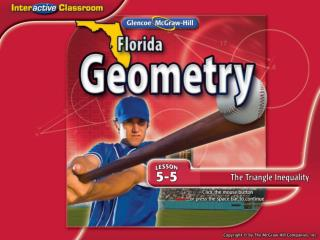DownloadDownload PresentationSplash Screen

# Splash Screen

Télécharger la présentation## Splash Screen

- - - - - - - - - - - - - - - - - - - - - - - - - - - E N D - - - - - - - - - - - - - - - - - - - - - - - - - - -
##### Presentation Transcript

1. Splash Screen

2. Five-Minute Check (over Lesson 5–4) NGSSS Then/Now Theorem 5.11: Triangle Inequality Theorem Example 1: Identify Possible Triangles Given Side Lengths Example 2: Standardized Test Example Example 3: Real-World Example: Proof Using Triangle Inequality Theorem Lesson Menu

3. A B C D A. ABC is a right triangle. B. A = D C.AB = DE D.ΔABC / ΔDEF ___ ___  State the assumption you would make to start an indirect proof of the statement.ΔABC ΔDEF 5-Minute Check 1

4. A B C D State the assumption you would make to start an indirect proof of the statement.RS is an angle bisector. ___ ___ A.RS is a perpendicular bisector. B.RS is not an angle bisector. C.R is the midpoint of ST. D. R = 90° ___ ___ 5-Minute Check 2

5. A B C D State the assumption you would make to start an indirect proof of the statement.X is a right angle. A.X is a not a right angle. B.X < 90° C.X > 90° D.X = 90° 5-Minute Check 3

6. A B C D State the assumption you would make to start an indirect proof of the statement.If 4x – 3 ≤ 9, then x ≤ 3. A.4x – 3 ≤ 9 B.x > 3 C.x > 1 D.4x ≤ 6 5-Minute Check 4

7. A B C D A.ΔMNO is a right triangle. B.ΔMNO is an isosceles triangle. C.ΔMNO is not an equilateral triangle. D.MN = NO = MO ___ ___ ___ State the assumption you would make to start an indirect proof of the statement.ΔMNO is an equilateral triangle. 5-Minute Check 5

8. A B C D Which statement is a contradiction to the statement that AB CD? ___ ___ A.AB = CD B.AB > CD C.CD  AB D.AB ≤CD ___ ___ 5-Minute Check 6

9. LA.1112.1.6.2The student will listen to, read, and discuss familiar and conceptually challenging text. MA.912.G.4.7 Apply the inequality theorems: triangle inequality, inequality in one triangle, and the Hinge Theorem. NGSSS

10. You recognized and applied properties of inequalities to the relationships between the angles and sides of a triangle. (Lesson 5–3) • Use the Triangle Inequality Theorem to identify possible triangles. • Prove triangle relationships using the Triangle Inequality Theorem. Then/Now

11. Concept

12. A. Is it possible to form a triangle with the given side lengths of 6 , 6 , and 14 ? If not, explain why not. 1 1 1 __ __ __ 2 2 2 Answer: Identify Possible Triangles Given Side Lengths Check each inequality.  X Example 1

13. Check each inequality. 6.8 + 7.2 > 5.1 7.2 + 5.1 > 6.8 6.8 + 5.1 > 7.2 14 > 5.1 12.3 > 6.8 11.9 > 7.2 Since the sum of all pairs of side lengths are greater than the third side length, sides with lengths 6.8, 7.2, and 5.1 will form a triangle.    Identify Possible Triangles Given Side Lengths B. Is it possible to form a triangle with the given side lengths of 6.8, 7.2, 5.1? If not, explain why not. Answer: yes Example 1

14. A B A. yes B. no Example 1

15. A B B. Is it possible to form a triangle given the side lengths 4.8, 12.2, and 15.1? A. yes B. no Example 1

16. In ΔPQR, PQ = 7.2 and QR = 5.2. Which measure cannot be PR? A 7 B 9 C 11 D 13 Example 2

17. Read the Test Item You need to determine which value is not valid. Solve the Test Item Solve each inequality to determine the range of values for PR. Example 2

18. Graph the inequalities on the same number line. Notice that n > –2 is always true for any whole number measure for x. Combining the two remaining inequalities, the range of values that fit both inequalities is n > 2 and n < 12.4 which can be written as 2 < n < 12.4. Example 2

19. Examine the answer choices. The only value that does not satisfy the compound inequality is 13 since 13 is greater than 12.4. Thus, the answer is choice D. Answer: D Example 2

20. A B C D In ΔXYZ, XY = 6, and YZ = 9. Which measure cannot be XZ? A. 4 B. 9 C. 12 D. 16 Example 2

21. Proof Using Triangle Inequality Theorem TRAVEL The towns of Jefferson, Kingston, and Newbury are shown in the map below. Prove that driving first from Jefferson to Kingston and then Kingston to Newbury is a greater distance than driving from Jefferson to Newbury. Example 3

22. Proof Using Triangle Inequality Theorem Abbreviating the vertices as J, K, and N: JK represents the side of the triangle from Jefferson to Kingstown; KN represents the side of the triangle from Kingston to Newbury; and JN the side of the triangle from Jefferson to Newbury. Answer:By the Triangle Inequality Theorem, JK + KN > JN. Therefore, driving from Jefferson to Kingston and then Kingston to Newbury is a greater distance than driving from Jefferson to Newbury. Example 3

23. A B Jacinda is trying to run errands around town. She thinks it is a longer trip to drive to the cleaners and then to the grocery store, than to the grocery store alone. Determine whether Jacinda is right or wrong. A. Jacinda is correct, HC + CG > HG. B. Jacinda is not correct, HC + CG < HG. Example 3

24. End of the Lesson